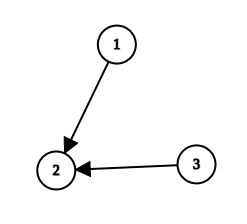# Treehouse May long challenge test cases 1 and 2

I did consider the structure of tree but got WA in test case 1 and 2
because I made directed graph. But when i converted it to undirected
graph(leaving everything else unchanged), case 1 and 2 passed. Is there any
problem with cases 1 and 2 because this isn’t suppose to happen. If you
make undirected graph then you have to anyway skip the edge to parent. If you make directed graph then you don’t
have to do that, that is why I made directed graph. I have tried 20-30 manual test cases, for all of them my directed graph version is giving correct answer. It is only failing for cases 1 and 2. Converting it to undirected graph, solves cases 1 and 2. Please clarify this.
These are my solutions:-
Directed graph: CodeChef: Practical coding for everyone
Undirected graph: CodeChef: Practical coding for everyone

Given that it is a Tree, An edge is not directed (unless specified). Now, the inputs are undirected edges. An edge between two vertices `a` and `b` can be given in two ways.

``````a b
``````

or

``````b a
``````
• If you take it as a directed graph, the first input gives you an edge like `a -> b`, while the second one gives an edge like `b -> a`.

It is also given that the tree is rooted at 1 and you start assigning values from the root node. A simple graph where two different solutions are possible for a directed graph.

``````3
1 2
2 3
``````

The given Graph is:``````3
1 2
3 2
``````

The same graph given differently:Now, find how the logic behind your code works for the same tree given in two ways.

If you take it as an undirected graph, the input format doesn’t matter.

1 Like

@suman_18733097 Wow! really just wow. Thanks a lot.

You didn’t properly make the tree directed as @suman_18733097 said…
I also needed to make the tree directed for a problem (it made implementation handy for me). Look at the direct function in my code it makes the tree directed with the root at the value initially passed to it. Observe we don’t care about root in an undirected tree but we do care in an directed one.)